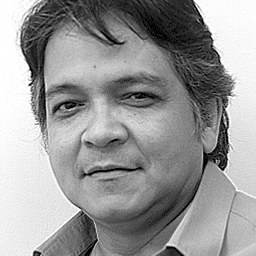💬 👋 We’re always here. Join our Discord to connect with other students 24/7, any time, night or day.Join Here!# Oswaldo J.

Université Bordeaux I

## Biography

I am a mathematician graduated at Central University of Venezuela (1981-1986). At this same University, I got a MSc degree in Mathematics (1986-1989). Then, I got a PhD (Doctorate) in Mechanical Engineering at Université de Bordeaux I, in France (1991-1995). I have worked at the Venezuelan Petroleum Company for almost 15 years (1989-2003), doing applied mathematical and numerical modelling, both for petroleum reservoirs and sedimentary basins. I have also worked for more than 16 years at the University, teaching numerical analysis, differential equations and scientific computing. I have been involved intensively in the mathematical and numerical modelling of porous media phenomena, through the numerical solution of ordinary and partial differential equations, using several numerical methods such as finite differences, finite elements, finite volumes, mimetics, among others. I have also been greatly involved in the process of scientific programming using some computer languages such as C, C++, Fortran, Python, Visual Basic, Matlab, Java, and others. I like solving complex problems by using mathematical, numerical and algorithmic tools.

## Education

Phd Mechanical Engineering
Université Bordeaux I
MS Mathematics
BS Mathematics

## Topics Covered

Functions
Derivatives
Differentiation
Vector Functions
Partial Derivatives
Multiple Integrals
Vector Calculus
Limits
Sequences
Series
Series Tests
Differential Equations
Applications of the Derivative
Integrals
Integration
Integration Techniques
Power Series
Powers and Polynomial
Trig Integrals
Trig Substitution
Matrices
Introduction to Matrices
Equations and Inequalities
Functions
Linear Functions
Polar Coordinates
An Introduction to Geometry
Introduction to Conic Section
Continuous Functions
The Language of Algebra
Complex Numbers
Exponential and Logarithmic Functions
Polynomials
Rational Functions
Trigonometry
Graphs and Statistics
Parametric Equations
Applications of Integration
Introduction to Trigonometry
Systems of Equations and Inequalities
Algebra Topics That are Reviewed at the Start of the Semester
Functions of Several Variables
Foundations for Geometry
Geometry Basics
Right Triangles
Circles
Introduction to Conic Sections
Introduction to Sequences and Series
Introduction to Combinatorics and Probability
Volume
Vectors
1 2 3 4 5 ... 170